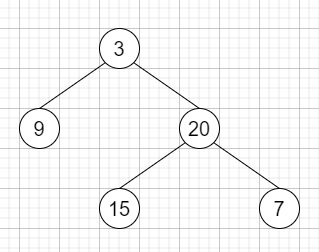# Binary Tree Vertical Order Traversal in C++

C++Server Side ProgrammingProgramming

Suppose there is a binary tree, we have to find the vertical order traversal of its nodes' values. If two nodes are in the same row and column, the order should be from left to right.

So, if the input is like,then the output will be [,[3,15],,]

To solve this, we will follow these steps −

• Define one map m

• Define a function solve(), this will take node, x initialize it with 0,

• if node is null, then −

• return

• solve(left of node, x - 1)

• solve(right of node, x + 1)

• insert value of node at the end of m[x]

• From the main method, do the following −

• if root is null, then −

• return {}

• Define one queue q

• insert { 0, root } into q

• insert value of node at the end of m

• while (q is not empty), do −

• sz := size of q

• while sz is non-zero, decrease sz in each iteration, do −

• curr := first element of q

• delete element from q

• node = second element of curr

• x := first element of curr

• if left of node is not null, then −

• insert { x - 1, left of node} into q

• left of node at the end of m[x - 1]

• if right of node is not null, then −

• insert { x - 1, right of node} into q

• right of node at the end of m[x - 1]

• Define one 2D array ret

• for each key-value pair 'it' of m do −

• insert value of it into ret

• return ret

## Example

Let us see the following implementation to get a better understanding −

Live Demo

#include <bits/stdc++.h>
using namespace std;
void print_vector(vector<vector<auto< > v){
cout << "[";
for(int i = 0; i<v.size(); i++){
cout << "[";
for(int j = 0; j <v[i].size(); j++){
cout << v[i][j] << ", ";
}
cout << "],";
}
cout << "]"<<endl;
}
class TreeNode{
public:
int val;
TreeNode *left, *right;
TreeNode(int data){
val = data;
left = NULL;
right = NULL;
}
};
void insert(TreeNode **root, int val){
queue<TreeNode*> q;
q.push(*root);
while(q.size()){
TreeNode *temp = q.front();
q.pop();
if(!temp->left){
if(val != NULL)
temp->left = new TreeNode(val);
else
temp->left = new TreeNode(0);
return;
}
else{
q.push(temp->left);
}
if(!temp->right){
if(val != NULL)
temp->right = new TreeNode(val);
else
temp->right = new TreeNode(0);
return;
}
else{
q.push(temp->right);
}
}
}
TreeNode *make_tree(vector<int< v){
TreeNode *root = new TreeNode(v);
for(int i = 1; i<v.size(); i++){
insert(&root, v[i]);
}
return root;
}
class Solution {
public:
map<int, vector<int< > m;
void solve(TreeNode* node, int x = 0){
if (!node || node->val == 0)
return;
solve(node->left, x - 1);
solve(node->right, x + 1);
m[x].push_back(node->val);
}
static bool cmp(vector<int<& a, vector<int<& b){
return a != b ? a < b : a < b;
}
vector<vector<int< > verticalOrder(TreeNode* root){
if (!root)
return {};
queue<pair > q;
q.push({ 0, root });
m.push_back(root->val);
while (!q.empty()) {
int sz = q.size();
while (sz--) {
pair<int, TreeNode*> curr = q.front();
q.pop();
TreeNode* node = curr.second;
int x = curr.first;
if (node->left && node->left->val != 0) {
q.push({ x - 1, node->left });
m[x - 1].push_back(node->left->val);
}
if (node->right && node->right->val != 0) {
q.push({ x + 1, node->right });
m[x + 1].push_back(node->right->val);
}
}
}
vector<vector<int< > ret;
map<int, vector<int< >::iterator it = m.begin();
while (it != m.end()) {
ret.push_back(it->second);
it++;
}
return ret;
}
};
main(){
Solution ob;
vector<int< v = {3,9,20,NULL,NULL,15,7};
TreeNode *root = make_tree(v);
print_vector(ob.verticalOrder(root));
}

## Input

{3,9,20,NULL,NULL,15,7}

## Output

[[9, ],[3, 15, ],[20, ],[7, ],]